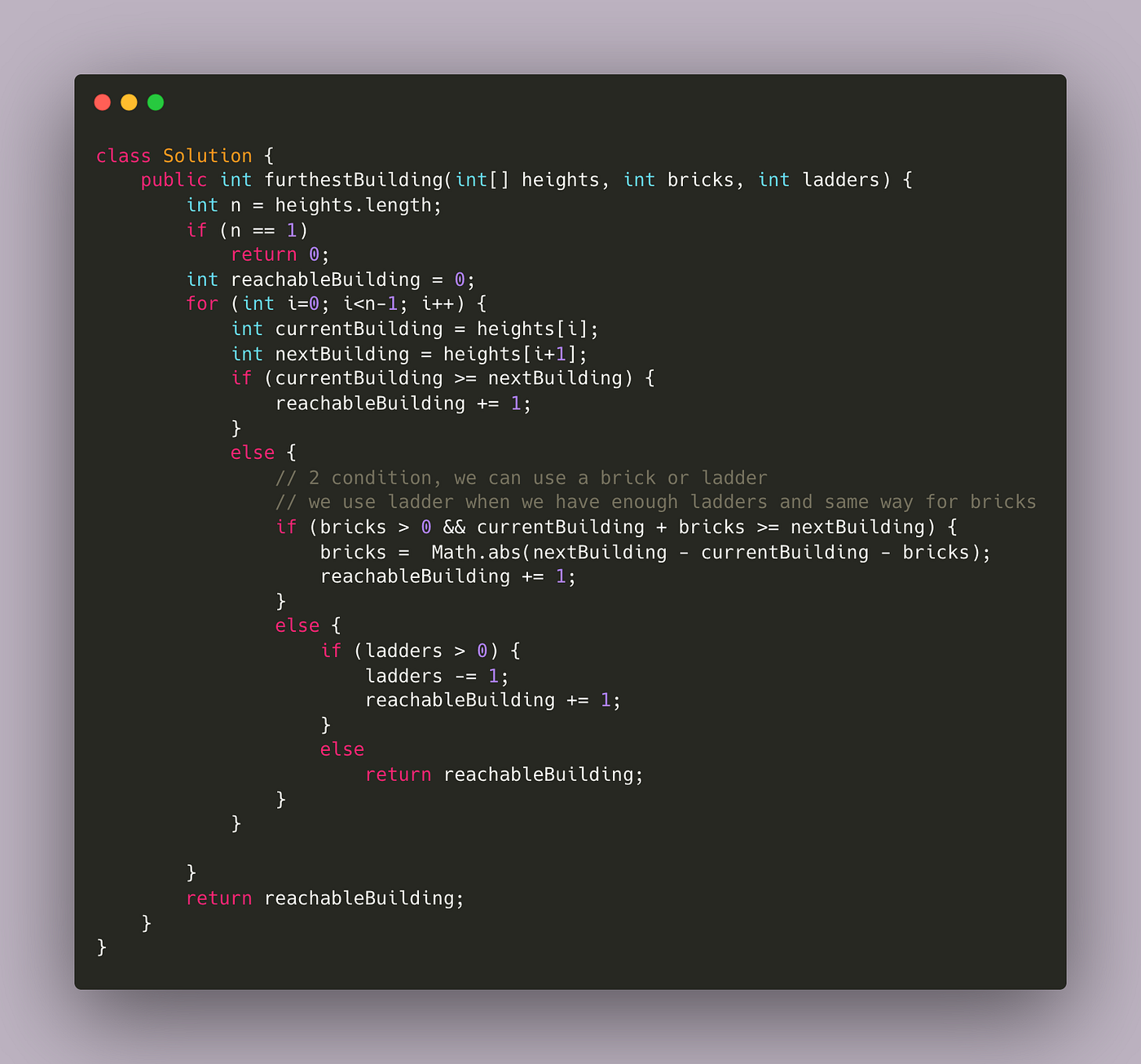# Problem Statement

You are given an integer array `heights` representing the heights of buildings, some `bricks`, and some `ladders`.

You start your journey from building `0` and move to the next building by possibly using bricks or ladders.

While moving from building `i` to building `i+1` (0-indexed),

• If the current building’s height is greater than or equal to the next building’s height, you do not need a ladder or bricks.
• If the current building’s height is less than the next building’s height, you can either use one ladder or `(h[i+1] - h[i])` bricks.

Return the furthest building index (0-indexed) you can reach if you use the given ladders and bricks optimally.

# Test Case

Example 1:

`Input: heights = [4,2,7,6,9,14,12], bricks = 5, ladders = 1Output: 4Explanation: Starting at building 0, you can follow these steps:- Go to building 1 without using ladders nor bricks since 4 >= 2.- Go to building 2 using 5 bricks. You must use either bricks or ladders because 2 < 7.- Go to building 3 without using ladders nor bricks since 7 >= 6.- Go to building 4 using your only ladder. You must use either bricks or ladders because 6 < 9.It is impossible to go beyond building 4 because you do not have any more bricks or ladders.`

Example 2:

`Input: heights = [4,12,2,7,3,18,20,3,19], bricks = 10, ladders = 2Output: 7`

Example 3:

`Input: heights = [14,3,19,3], bricks = 17, ladders = 0Output: 3`

# Constraints

• `1 <= heights.length <= 105`
• `1 <= heights[i] <= 106`
• `0 <= bricks <= 109`
• `0 <= ladders <= heights.length`

# Approach

There was a greedy solution which was accepted earlier but there were many changes in the test cases and it will not get accepted now. Sharing that approach.This was accepted before but not now as test cases are updated.

This approach just greedily consider whether we need to use bricks or ladder and update the result.

So our main aim of the problem is to find the farthest building that we can reach using bricks and ladders.

We can only use say `k` amount of ladders only. So our choice must be in such a way that we make use of this effectively. And for that we make use of heaps.

## Algorithm

1. Store the k height difference in the priority queue or heap(Min Heap).
2. If the height difference is greater than zero, just push it inside the heap.
3. If our heap size exceeds k, where k = number of ladders available, then it means we need to make use of bricks to make a move. So we have to pop out the smallest height difference.
4. Reduce the number of bricks with the smallest height difference which is available from the top of the heap.
5. If our number of bricks runs out, immediately return the index.
6. Else if we are able to reach the last building, return length — 1.

# Time and Space Complexity

We are making use of priority queue where we store k height difference and apply the sorting for k items. So time complexity will be O(nlogk) where n = length of array.

Space Complexity will be O(k) where k is the number of elements we are having inside priority queue.

# Code